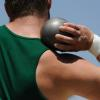You may also likeMaximum Scattering

Your data is a set of positive numbers. What is the maximum value that the standard deviation can take?David and Goliath

Does weight confer an advantage to shot putters?

Age 16 to 18 Challenge Level:

Most of the following solution was submitted by Farrukh Jawed of Beaconhouse, Lahore.

The number of candidates $n$ is 50 and the mean score $\overline x$ is 60. For there to be the maximum number of students who gained 100 marks the rest must score 0. Let $y$ be the number who gained 100 and z be the number who gained 0 marks. Then $(100y + 0z )/50 = 60$ so $y = 30$. Therefore, 30 students scored 100 and 20 students scored 0.

The standard deviation is given by $$\sigma^2 = {\sum(x_i-\overline x ^2)\over n} = {1\over n}\sum x_i^2 - \overline x^2 = 2400$$ so the standard deviation $\sigma = 20\sqrt 6 = 49.0$ to 3 s.f.

For a lower standard deviation it is not possible for so many students to score 100. Now suppose the standard deviation $\sigma = 8$. If $y$ candidates score 100 then, as the marks of the remaining 48 candidates contribute to the standard deviation, we know $$\sigma^2 > {40^2y\over 50},$$ so we know $40^2 y < 3200$ so $y< 2$.

If the standard deviation is a little higher, say 8.2. Then $40^2y< 50\times 8.2^2$ so $y< 2.10125$. As $y$ is a whole number it could be 2 (but not more than 2).

If 2 students got 100, and the mean is 60, the sum of all the marks is 3000 and the sum of the scores of the remaining 48 students is 2800. Then 2800/48 = 58.3333.... so one set of results might be 47 students getting 58 marks, the 48th getting 74 marks, and 49th and 50th getting 100 marks. In this case the standard deviation is : $$\sigma ^2 = (2\times 100^2 + 74^2 + 47\times 58^2)/50 - 60^2$$ which gives $\sigma = 8.4664$.

Another set of possible scores with a lower standard deviation, around 8.2 or less, requires all candidates who don't score 100 to get scores as near to 60 as possible. Suppose $q$ candidates score 59 and ($48-q)$ score 58 then $$59q + 58(48-q) =2800$$ so $q=16$. In this case 2 candidates score 100, 16 score 59 and 32 score 58. The standard deviation is given by $$\sigma^2 = {2\times 40^2 + 16\times 1^2 + 32\times 2^2 \over 50} = 66.88.$$ So the standard deviation is 8.178 to 3 d.p.

If the standard deviation $\sigma = 4\sqrt 2$, as we know that the number of candidates who score 100 is always less than $$50\sigma^2\over 40^2,$$ the number who could have scored 100 is less than $${50 \times 32\over 40^2} = 1$$ so it is impossible for any of the candidates to have scored 100.